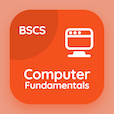Business Mathematics Quiz PDF - Complete

# Linear Functions in Maths Quiz Questions Online p. 7

Learn Linear Functions in Maths quiz questions and answers, linear functions in maths MCQ with answers PDF 7 to study Business Mathematics course online. Linear Function Applications trivia questions, linear functions in maths Multiple Choice Questions (MCQ) for online college degrees. "Linear Functions in Maths Quiz" PDF Book: matrix operations, simplex computer solutions, simplex preliminaries, simplex methods, linear functions in maths test prep for online courses for business management degree.

"In the depreciation function V = ƒ(t) then the t is" MCQ PDF: time from purchase date, interest value, purchased value, and sold value for business management degree online. Study linear function applications questions and answers to improve problem solving skills for online BS business administration.

## Quiz on Linear Functions in Maths MCQs

MCQ: In the depreciation function V = ƒ(t) then the t is

interest value
time from purchase date
purchased value
sold value

MCQ: In simplex method, the feasible basic solution must satisfy the

non-negativity constraint
negativity constraint
basic constraint
common constraint

MCQ: The column in the simplex initial table used to represent new basic variable is classified as

column variable
key column
key row
row variable

MCQ: The determination of effects of parameters set other than original formulation before the analysis is classified as

parameter analysis
original analysis
sensitivity analysis
formulation analysis

MCQ: The value of the determinant is computed by adding multiples of one column to

another column
another matrix
another dimension
another row

### More Quizzes from Business Mathematics Course